## Combinatorics Cheat Sheet

In each Cheat Sheet, I’ll cover, as succinctly as possible, every rule you absolutely must know to solve problems in a single area found on standardized tests.

Learning these rules isn’t a substitute for developing higher-order problem-solving and strategic thinking skills; rather, it’s a necessary precondition and foundation for all of that strategizing to take place. This Cheat Sheet lists the minimum requirements to get your foot in the door. It’s the price of admission.

If you’re taking any test involving combinatorics, here’s what you need to know:

Combinatorics Basics:

Factorials

For any positive integer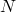,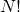means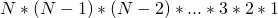.

Factorials to know:

•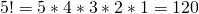•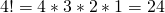•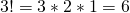•••The Basic Counting Principle

• “And” means MULTIPLY
• “Or” means ADD

Two Key Questions

Givenitems and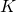picks, ask the following two questions:

• 1.  Is there replacement? (In other words, “can an item be reused once picked?”)
• Yes: Use the basic counting principle
• No: Move on to question 2.
• 2.  Does order matter?
• Yes: Permutation. Use the basic counting principle (the result will be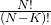)
• No: Combination. Use the combination formula:• Combinations to know (true for any):
••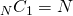••• Bonus: For any,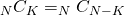.
• Bonus:For a given value of, the sum of all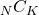is.
• (e.g.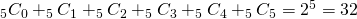)

Common Twists:

Restrictions: Calculate the total number of possibilities, ignoring the restriction. Subtract the number of “bad” options (those that violate the restriction).

Grouping: Calculate the number of ways to arrange items within each group separately; then calculate the number of ways in which the groups can be arranged relative to one another; multiply the results.

Duplicates: Calculate the permutation as though each item were distinct (the result will be). Divide the result by the factorial of the number of each type of duplicate (giving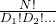).

Circular Arrangements: “One item free.” A circular arrangement ofitems will result inoptions. (A linear arrangement ofitems would giveoptions.)

Tags: , , , , , ,

### Comments

One Response to “Combinatorics Cheat Sheet”
1.Debo Aderibigbe says:

Anthony, these are great! I would make a couple of suggestions to make this even more helpful as a reference sheet:

1. Separate the example problems from the reference sheet themselves and just link them at the bottom, either to a blog post or a new page – and instead, near each reference, put a SMALL example next to the reference. (for Duplication, show letters repeating in a simple example, for Circular, show a table of 4 people, etc.) Just so there is some context for the references, and so that people can go to the examples when the feel ready to take then on.

2. I think in your first example problem, the explanation could be a bit cleaner towards the end. Point back to the fact alternation is caused because Vowels and Consonants can’t be back to back, not vice versa. And because with alternation, you can only start in two ways (vowel first or consonant first), that gives you two WAYS to group.

THEN explain that you can either view those groupings as and AND to the problem (two times the groupings of 48) or an OR to the problem (48 ways to group vowels first OR 48 ways to group Consonants first) and then you have your answer.